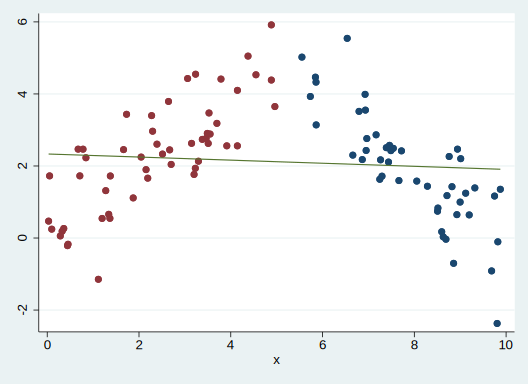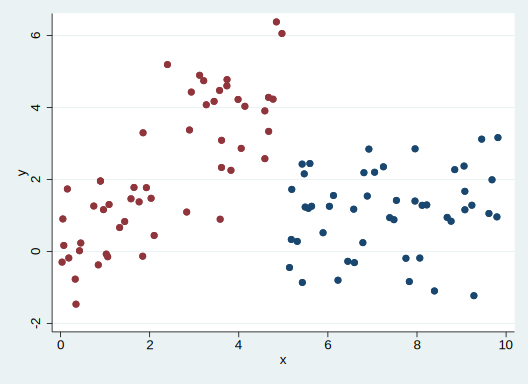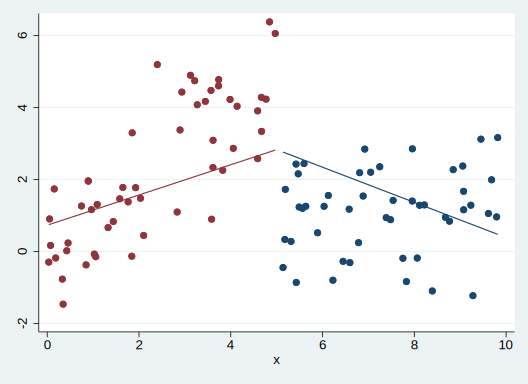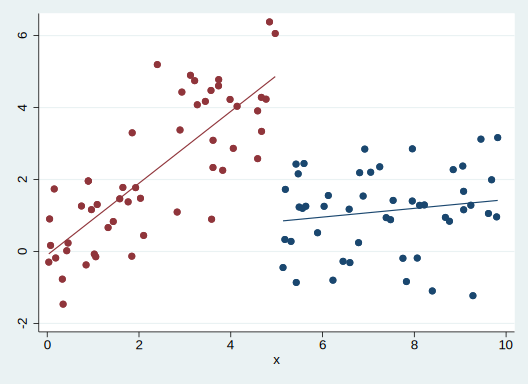Linear splines are sometimes used when looking at interrupted time series models. For example, consider the scatter plot below.The slope amongst the red points (`x < 5`) is clearly different from the slope amongst the blue points (`x > 5`). The best fit line fails to capture this at all.

Imagine that `x` is time, and at `x = 5`, some intervention took place. The goal is to capture the change in slope that occurs after the intervention. One easy approach would be to fit separate pre and post models, and test for equality of coefficients. However, we can also address this with a single model.

A linear spline model (as fit by Stata’s `mkspline`) can capture that change in trend. Including an indicator for pre/post even allows a discontinuity at `x = 5` instead of the typical continuous spline. However, splines can be harder to interpret and more complicated to work with. This document will demonstrate that an interaction model is equivalent to the linear spline model, and with a simple re-scaling, easier to interpret.

## Data generation

Let’s create a slightly more general data set where there is a “jump” (discontinuity) at intervention in addition to the change in trend.

``````. clear

. set obs 100
number of observations (_N) was 0, now 100

. gen x = runiform(0, 10)

. sort x // To ease plotting later

. gen z = x > 5

. gen y = x + z - x*z + rnormal()

. twoway (scatter y x if z == 1) (scatter y x if z == 0), legend(off)

``````Now there’s a drop of around 4 at the intervention addition to a flattening of the slope.

# Obtain pre and post slopes

For comparison purposes, let’s obtain the slopes in each time period.

``````. reg y x if z == 0

Source |       SS           df       MS      Number of obs   =        52
-------------+----------------------------------   F(1, 50)        =     89.11
Model |  125.414733         1  125.414733   Prob > F        =    0.0000
Residual |  70.3714084        50  1.40742817   R-squared       =    0.6406
Total |  195.786141        51  3.83894395   Root MSE        =    1.1864

------------------------------------------------------------------------------
y |      Coef.   Std. Err.      t    P>|t|     [95% Conf. Interval]
-------------+----------------------------------------------------------------
x |   .9983998   .1057653     9.44   0.000     .7859639    1.210836
_cons |  -.1003115   .2979778    -0.34   0.738    -.6988175    .4981945
------------------------------------------------------------------------------

. local preslope = _b[x]

. reg y x if z == 1

Source |       SS           df       MS      Number of obs   =        48
-------------+----------------------------------   F(1, 46)        =      1.18
Model |  1.54812546         1  1.54812546   Prob > F        =    0.2832
Residual |  60.4075857        46  1.31320838   R-squared       =    0.0250
Total |  61.9557112        47  1.31820662   Root MSE        =     1.146

------------------------------------------------------------------------------
y |      Coef.   Std. Err.      t    P>|t|     [95% Conf. Interval]
-------------+----------------------------------------------------------------
x |   .1214053   .1118153     1.09   0.283    -.1036673    .3464779
_cons |   .2272667   .8366968     0.27   0.787    -1.456917     1.91145
------------------------------------------------------------------------------

. local postslope = _b[x]

``````

So the pre slope is 0.998 and the post slope is 0.121. Their difference is -0.877.

# Spline version

The “intervention” takes place at `x = 5`, so let’s create the spline with a knot there.

``````. mkspline x0 5 x1 = x, marginal

``````

With the `marginal` option, `x0`’s coefficient will represent the pre-intervention slop and `x1`’s coefficient the difference between the pre- and post-intervention slopes (similar to an interaction). Without `marginal`, `x1`’s coefficient is the post-intervention slope. Note that this will not change the model, but is a simple reparameterization.

## Spline Model 1 - Continuous at intervention

First, we’ll predict `y` using only the splines. This forces a continuity at intervention.

``````. reg y x0 x1

Source |       SS           df       MS      Number of obs   =       100
-------------+----------------------------------   F(2, 97)        =      9.48
Model |  47.3187332         2  23.6593666   Prob > F        =    0.0002
Residual |  242.134186        97  2.49622872   R-squared       =    0.1635
Total |  289.452919        99  2.92376686   Root MSE        =    1.5799

------------------------------------------------------------------------------
y |      Coef.   Std. Err.      t    P>|t|     [95% Conf. Interval]
-------------+----------------------------------------------------------------
x0 |   .4201304   .1111038     3.78   0.000     .1996201    .6406408
x1 |  -.9088959   .2087659    -4.35   0.000    -1.323238   -.4945534
_cons |   .7302661   .3768498     1.94   0.056    -.0176763    1.478209
------------------------------------------------------------------------------

. est store spline1

. predict y_spline1
(option xb assumed; fitted values)

. twoway (scatter y x if z == 1) (scatter y x if z == 0) ///
>        (line y_spline1 x if z == 1, lcolor(navy)) ///
>        (line y_spline1 x if z == 0, lcolor(maroon)), ///
>          legend(off)

``````The continuity1 at `x = 5` makes this a poor fit.

## Spline Model 2 - Discontinuous at intervention

Simply adding `z` to the model will allow a discontinuity.

``````. reg y x0 x1 z

Source |       SS           df       MS      Number of obs   =       100
-------------+----------------------------------   F(3, 96)        =     38.83
Model |  158.673925         3  52.8913084   Prob > F        =    0.0000
Residual |  130.778994        96  1.36228119   R-squared       =    0.5482
Total |  289.452919        99  2.92376686   Root MSE        =    1.1672

------------------------------------------------------------------------------
y |      Coef.   Std. Err.      t    P>|t|     [95% Conf. Interval]
-------------+----------------------------------------------------------------
x0 |   .9983998   .1040552     9.59   0.000      .791852    1.204948
x1 |  -.8769945   .1542639    -5.69   0.000    -1.183206   -.5707831
z |  -4.057394   .4487716    -9.04   0.000    -4.948199    -3.16659
_cons |  -.1003115   .2931596    -0.34   0.733    -.6822287    .4816058
------------------------------------------------------------------------------

. est store spline2

. predict y_spline2
(option xb assumed; fitted values)

. twoway (scatter y x if z == 1) (scatter y x if z == 0) ///
>        (line y_spline2 x if z == 1, lcolor(navy)) ///
>        (line y_spline2 x if z == 0, lcolor(maroon)), ///
>          legend(off)

``````We capture the model much better here. Note that the coefficient on `x0` is the marginal slope we obtained before and `x1` is the difference between the slopes.

Additionally (and one of the major benefits that linear spline proponents point to) is that the coefficient on `z`, -4.06, captures the drop that occurs at `x = 5` - in the pre-period, the best fit line is approaching ~5, and in the post-period, the best fit line is approaching ~1.

## Without `marginal`

Let’s generate the splines without the `marginal` option to show the results are the same.

``````. mkspline x0a 5 x1a = x

``````
``````. reg y x0a x1a z

Source |       SS           df       MS      Number of obs   =       100
-------------+----------------------------------   F(3, 96)        =     38.83
Model |  158.673925         3  52.8913084   Prob > F        =    0.0000
Residual |  130.778994        96  1.36228119   R-squared       =    0.5482
Total |  289.452919        99  2.92376686   Root MSE        =    1.1672

------------------------------------------------------------------------------
y |      Coef.   Std. Err.      t    P>|t|     [95% Conf. Interval]
-------------+----------------------------------------------------------------
x0a |   .9983998   .1040552     9.59   0.000      .791852    1.204948
x1a |   .1214053   .1138854     1.07   0.289    -.1046554    .3474659
z |  -4.057394   .4487716    -9.04   0.000    -4.948199    -3.16659
_cons |  -.1003115   .2931596    -0.34   0.733    -.6822287    .4816058
------------------------------------------------------------------------------

. est store spline3

. predict y_spline3
(option xb assumed; fitted values)

. twoway (scatter y x if z == 1) (scatter y x if z == 0) ///
>        (line y_spline3 x if z == 1, lcolor(navy)) ///
>        (line y_spline3 x if z == 0, lcolor(maroon)), ///
>          legend(off)

``````The model is identical, but the coefficient on `x1a` is now the slope in the post period.

# Interaction model

If we fit a simple interaction model here, we obtain the same model.

``````. reg y c.x##c.z

Source |       SS           df       MS      Number of obs   =       100
-------------+----------------------------------   F(3, 96)        =     38.83
Model |  158.673925         3  52.8913084   Prob > F        =    0.0000
Residual |  130.778994        96  1.36228119   R-squared       =    0.5482
Total |  289.452919        99  2.92376686   Root MSE        =    1.1672

------------------------------------------------------------------------------
y |      Coef.   Std. Err.      t    P>|t|     [95% Conf. Interval]
-------------+----------------------------------------------------------------
x |   .9983998   .1040552     9.59   0.000      .791852    1.204948
z |   .3275782   .9012017     0.36   0.717    -1.461293    2.116449
|
c.x#c.z |  -.8769945   .1542639    -5.69   0.000    -1.183206   -.5707831
|
_cons |  -.1003115   .2931596    -0.34   0.733    -.6822287    .4816058
------------------------------------------------------------------------------

. predict y_naive
(option xb assumed; fitted values)

. twoway (scatter y x if z == 1) (scatter y x if z == 0) ///
>        (line y_naive x if z == 1, lcolor(navy)) ///
>        (line y_naive x if z == 0, lcolor(maroon)), ///
>          legend(off)

``````